# 5.10. Exercises¶

1. Write a program that prints `We like Python's turtles!` 100 times.

1. Turtle objects have methods and attributes. For example, a turtle has a position and when you move the turtle forward, the position changes. Think about the other methods shown in the Summary of Turtle Methods page. Which attibutes, if any, does each method relate to? Does the method change the attribute?

1. Use `for` loops to make a turtle draw these regular polygons (regular means all sides the same lengths, all angles the same):

• An equilateral triangle

• A square

• A hexagon (six sides)

• An octagon (eight sides)

```# draw an equilateral triangle
import turtle

wn = turtle.Screen()
norvig = turtle.Turtle()

for i in range(3):
norvig.forward(100)

# the angle of each vertice of a regular polygon
# is 360 divided by the number of sides
norvig.left(360/3)

wn.exitonclick()
```
```# draw a square
import turtle

wn = turtle.Screen()
kurzweil = turtle.Turtle()

for i in range(4):
kurzweil.forward(100)
kurzweil.left(360/4)

wn.exitonclick()
```
```# draw a hexagon
import turtle

wn = turtle.Screen()
dijkstra = turtle.Turtle()

for i in range(6):
dijkstra.forward(100)
dijkstra.left(360/6)

wn.exitonclick()
```
```# draw an octogon
import turtle

wn = turtle.Screen()
knuth = turtle.Turtle()

for i in range(8):
knuth.forward(75)
knuth.left(360/8)

wn.exitonclick()
```
1. Write a program to draw a shape like this:1. Write a program to draw a face of a clock that looks something like this: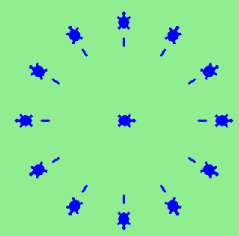1. Write a program to draw some kind of picture. Be creative and experiment with the turtle methods.

1. Create a turtle and assign it to a variable. When you print its type, what do you get?

## 5.10.1. Contributed Exercises¶

Write a program that prints `I love Python!` 15 times.
Turtle exercise:Ask the user for a side length and then draw a triangle with a turtle.
Turtle exercise: ask the user for a side length and then draw an octagon (8-sided) with a turtle.
Consider the program below which prints `I love Python` 10 times. To avoid hard-coding, change this program so that it asks a user for an input that specifies how many times `I love Python` should be printed. So if the user says `3` times, the program will print it `3` times. It should work for any input without you manually changing the code.

Write a function to compute pi to 5000 places

Q-1: After completing the reading, what concepts are still unclear to you? If nothing is unclear, what did you find most interesting?

Write a turtle program that draws the letters LLL in green. In order to get credit you must use one loop repeating the same thing three times but at a different location, one for each L.

Use split (by the empty space) and join (by the “-“) to transform the string “Once upon a time” into “Once-upon-a-time”. Print your result. Write it all in one line.

Write a turtle program that asks the user “how many sides?”. If the number of sides is even, draw two regular polygon with that many sides, one inside the other. If the number of sides is odd, draw two regular polygons with that many sides, side by side.

Use the turtle module to draw an L. The side of the L should be 100 long and the width of the L should be 75 long. The top left should start at \(x=-100\), \(y=100\).

Use the turtle module to create a turtle `tuck`. Use tuck to draw a box with it’s center at (\(x=0\), \(y=0\)). The width of the box should be 100 and the height of the box should be 200.

This is our shared activecode window for today’s class. We’ll be coding a DVD bouncing ball with turtles

Write code to ask the user for his/her name. Then use Turtle to draw “Hi!” or “Hello!” at random (not the quotes; make it look EXACTLY the same) followed by a PRINT OUT (not a drawing) of the name. Say t is the name of your turtle; use t.write(name) to write the name after Hi! or Hello!.

Write a program to draw some kind of picture. Be creative and experiment with the turtle methods.

10 points for creativity

10 points for using a for loop

10 points for using multiple colors

10 points for keeping picture inside of the window

10 points for using at least 4 turtle methods

Create a turtle and assign it to a variable. Print the type of your turtle and see what you get.

Reorganise the code so the turtle draws a square

config values (conf.py):

• parsons_div_class - custom CSS class of the component’s outermost div

Write a Python program that prompts the user for the number of sides of a shape, and the length of a side. Your program should then use a Python turtle to draw a regular polygon with that many sides, each side of the given length. I have started the program for you.

Use the turtle module to create a turtle `tuck`. Use tuck to draw a box with it’s center at (\(x=0\), \(y=0\)). The width of the box should be 100 and the height of the box should be 200.

Use the turtle module to draw a Z in green. The top and bottom of the Z should each be 50 long and the diagonal of the Z should be 75 long.

Write a program that uses a for loop to print `I like Python!` 100 times (see Chapter 5 to see how a for loop is used). Please don’t write manually this sentence 100 times :) It will take a while. If you use a for loop, this code is only 3 lines.

Buatlah sebuah program untuk menghasilkan output turtle berbentuk bintang

Write a program that prints `We like Python's turtles!` 100 times.

Complete the code below to draw turn what we currently have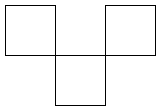into a T-tetromino: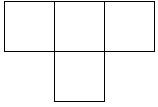This one will be manually graded by the TAs.

Overview: Write a Python program to draw a closed regular polygon, given the number of sides and the side length.

Details: Begin by prompting the user to enter the number of sides they would like for their shape. Store this in variable `numSides`. Then, prompt the user for the length of a side (in a regular polygon, all sides are of the same length). Store this in variable `sideLength`.

Once you have these values, use a Python turtle to draw a shape that matches those parameters.

For example, if a user answered `4` and `30`, respectively, to your prompts, then your program would draw a square with sides of length 30. If instead the user responded with `6` and `25`, then your program would draw a hexagon with sides of length 25.

Once you have completed and tested your program, construct your solution explanation video and submit it (the video) in response to the HW02 assignment on the course Canvas page. (See Canvas for more details.)

Arrange the code below so that the shape shown in the linked image is drawn: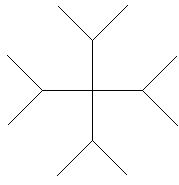Arrange the code below so that the shape shown in the image is drawn.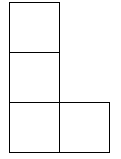Use `for` loops to make a turtle draw these regular polygons (regular means all sides the same lengths, all angles the same):

• An equilateral triangle

• A square

• A hexagon (six sides)

• An octagon (eight sides)

Write a turtle program that draws the letter A in green. In order to get credit you must use one loop repeating the same thing three times but at a different location, one for each L.

Write a turtle program that asks the user “how many sides?”. If the number of sides is even, draw two regular polygon with that many sides, one inside the other. If the number of sides is odd, draw three regular polygons with that many sides, one inside the other.

Write code to transform “hello” into “Hello”. Print your result.

Write a turtle program that draws a staircase with 5 steps. In order to get full credit you must use a loop.

Run the following code to see what is drawn. Then modify the code so that

1. the circles on the top are solid red, and

2. there are no lines connecting any of the circles.

Write a program that prints `Python programming is fun!` 95 times (each on a separate line).

Do not hardcode.

Write a code which uses the turtle module to draw a stick figure

Write a program by completing the following:

1. Instantiate a turtle object. Set the turtle’s speed to 10 and pensize to 5.

2. Generate a random integer between 0 and 10 (inclusive) and store it in a variable called `num`.

3. If `num` is less than 5, color the turtle blue and turn it left 60 degrees. If `num` is greater than 5, color the turtle magenta and turn it right 60 degrees. If `num` is equal to 5, color the turtle black.

4. Move the turtle forward a distance 15.

5. Repeat steps 2-4 so that the turtle walks a total of 50 times.

Write a program by completing the following:

1. Instantiate turtle object.

2. Generate a random integer between 20 and 30 (inclusive) and store it in a variable called `num`.

3. If `num` is even, color the turtle green, turn it left an angle `num` and move it forward a distance `num`. If `num` is odd, color the turtle orange, turn it right an angle `num` and move it backward a distance `num`.

4. Repeat steps 2 and 3 so that the turtle walks a total of 30 times.